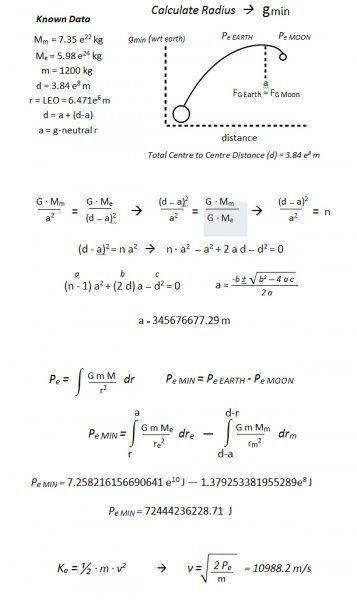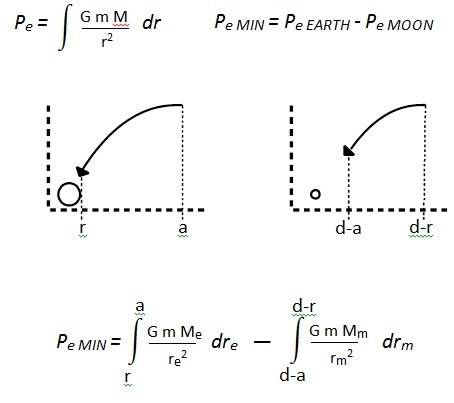# Gravitational Potential Energy of a fall

In this case the height of the fall is far to high above earth to be use PE=mgh. So I'm trying to integrate the inverse square gravitational force over distance.

##\int \frac{GmM_e}{r^2}dr##

So I'm confused with respect to another object, specifically the moon, if the max height of the fall is from the point where Fg Earth = Fg Moon.

How do I expand the integral... I think its one of these but I'm not sure which one...

Let
h = Height of the fall which is the radius where Fg Earth = Fg Moon.
b = Earth's total distance from the moon - h
n = Bottom of the fall in LEO.
m = Mass of Falling Object.
Me = Mass of the Moon.
Me = Mass of the Earth.

I feel like I shouldn't even include the moon in the integral at all because the fall is up to the point where gmoon < gearth. So I think it might be...

##\frac{-GmM_e}{h} - \frac{-GmM_e}{n}##

But I was told it should be and the moon should be included.

##\frac{-GmM_e}{h} + \frac{-GmM_m}{b}##

I'm still missing an element in understanding the math which is okay because I'm a level below this in school, but I like to stay ahead so if you could please explain which answer is right and why, I'd be very grateful?

Last edited:

mfb
Mentor
Even if the force from the moon is smaller, it is still there (assuming you are right between moon and earth) and relevant.

The two formulas are answers to completely different questions. What do you want to calculate?

Even if the force from the moon is smaller, it is still there (assuming you are right between moon and earth) and relevant.

The two formulas are answers to completely different questions. What do you want to calculate?

I want to calculate the minimum velocity required to make it to the Fg moon = Fg earth point (which that distance is the variable h) from LEO orbit (which that distance is the variable n)... Distance is obviously the measure of how far from the center of earth. Where there is also a variable d being the center to center distance from earth to the moon.

Last edited:
mfb
Mentor
Minimum velocity from (at?) LEO to do what?
In the way you use it, n looks like a distance, not like a velocity.

Minimum velocity from (at?) LEO to do what?
In the way you use it, n looks like a distance, not like a velocity.
I edited that sorry.

haruspex
Homework Helper
Gold Member
Have you calculated the point at which the forces balance?
What work has to be done against Earth's gravity to get there?
What work is done by the moon's gravity in the process?

•mfb
To get h in FG earth = FG moon

I did the algebra to get the quadratic on h... ##\frac{GM_e}{h^2} = \frac{GM_m}{(d-h)^2}## which was simple enough...

So then If I understand correctly, ##\frac{-GmM_e}{h} - \frac{-GmM_e}{n}## is the PE wrt the earth on the object between these distances in the denominators?

##\frac{-GmM_m}{d-h} - \frac{-GmM_m}{d-n}## Would be the PE in the craft wrt the moon?

Then when you do the algebra with all the instances of PE the ship experiences you get left with the integral expansion of...

##\frac{-GmM_e}{h} + \frac{-GmM_m}{d-h}## which is the value of PE at the height of d to d-h if the object falls over the gravitational hill back down to earth to the radius at n in LEO ?[/QUOTE]

Last edited:
mfb
Mentor
b=d?
So then If I understand correctly, ##\frac{-GmM_e}{h} - \frac{-GmM_e}{n}## is the PE wrt the earth on the object between these distances in the denominators?

##\frac{-GmM_m}{d-h} - \frac{-GmM_m}{d-n}## Would be the PE in the craft wrt the moon?
Looks good.
Add both and you get the total difference in potential energy between the two positions.

##\frac{-GmM_e}{h} + \frac{-GmM_m}{d-h}## which is the value of PE at the height of d to d-h if the object falls over the gravitational hill back down to earth to the radius at n in LEO ?
That is the potential energy at a distance h from earth. It has nothing to do with LEO.

haruspex
Homework Helper
Gold Member
##\frac{-GmM_m}{d-h} - \frac{-GmM_m}{d-n}## Would be the PE in the craft wrt the moon?
All looks good to here, but I expect that last term will be small enough to ignore.
Then when you do the algebra with all the instances of PE the ship experiences you get left with the integral expansion of...

##\frac{-GmM_e}{h} + \frac{-GmM_m}{d-h}## ?
how do you get that?

All looks good to here, but I expect that last term will be small enough to ignore.how do you get that?
How about this ? I put it in an image because it's quicker... The whole deal with factoring in the moon is really confusing me because this is out of my league, but I'm trying to comprehend it... I think this is getting closer but if I got this wrong still I'm really lost.The LEO variable r is because thats the starting distance where the object need to be going the initial velocity by in launch... I'm not factoring the ground into it because I figured I would separate the launch from the lunar injection shot since it's going to depend on thrust forces and variable mass with loss of fuel etc...

So if I launch from 6.371e6 m, by 6.471e6 m, I must be traveling the calculated initial velocity, this gives the object 100 km to accelerate the object to the initial velocity, while getting the mass down to 1200 kg, and also set it in the right direction.

That's why I think this should be the lower bound with respect to Earth for the potential energy, and the distance minus this LEO radius for the upper bound of the potential energy with respect to the moon?

But again, incorporating the moon is where I'm brain farting. The closer the object gets to the moon, the more the moon attracts it, even though the earth is attracting it the other way more.

So the first integral takes the PE with respect to earth from falling from the a distance to LEO without the moon in the picture at all. Then I subtract the potential energy with respect to falling to the moon between said distance integrated, but from the moons frame of reference.

I think this is right, because subtracting the work the moon does pulling the craft gives the initial work required without the help of the moon, and without subtracting the work the moon does, the initial velocity would be too fast because it's neglecting the help the moon is giving right?

With the y axis being the force of gravity (max @ origin) and x axis being distance (min @ origin), I guess I'm looking at it this way...Last edited:
haruspex
Homework Helper
Gold Member
I don't understand why you are doing any integration. In post #7 you gave a correct quadratic equation for determining h. You went on to provide correct expressions in terms of h for the PE changes, both wrt Earth and wrt Moon, as the object goes from Earth's surface to h. Why don't you just add those up for the total PE change and substitute in the value of h?

I don't understand why you are doing any integration. In post #7 you gave a correct quadratic equation for determining h. You went on to provide correct expressions in terms of h for the PE changes, both wrt Earth and wrt Moon, as the object goes from Earth's surface to h. Why don't you just add those up for the total PE change and substitute in the value of h?
How do you mean? I think thats what I did by subtracting the integral wrt earth from the integral wrt moon... The total PE of either system requires an integral? and in post 7 I had the integral expanded which you mentioned that the integral for PE with respect to the moon was correct.

I've basically subtracted the PE of a towards to the moon without earth...

r max = the total center to center distance minus the LEO radius at earth
to
r min = total center to center distance minus quadratic height

From the PE of a fall to earth without the moon...

r max = the quadratic height
to
r min = the LEO radius at earth

Which gave me the left over PE being the KE required to get from LEO at Earth to the quadratic height in the direction towards the moon...

I'm pretty sure this is right because the moon PE that I subtracted will be made up along the way by the gravitational work that the moon does pulling the object as it moves in the moon's direction.

Last edited:
haruspex
Homework Helper
Gold Member
In post #7 you wrote
To get h in FG earth = FG moon

I did the algebra to get the quadratic on h... ##\frac{GM_e}{h^2} = \frac{GM_m}{(d-h)^2}## which was simple enough...

So then If I understand correctly, ##\frac{-GmM_e}{h} - \frac{-GmM_e}{n}## is the PE wrt the earth on the object between these distances in the denominators?

##\frac{-GmM_m}{d-h} - \frac{-GmM_m}{d-n}## Would be the PE in the craft wrt the moon?
Up to this point, you made no mention of performing any integrals, so I presumed you were using the standard formula for gravitational PE. Yes, you can get it by integrating the force formula, but it's a very standard result.
From this point, you can get the answer without any further integration, agreed?

I believe that ##\frac{-GmM_e}{h} - \frac{-GmM_e}{n}## is the integral expansion of ##\int \frac{GmM_e}{r^2}dr## which the interval max = h, and min = n...

And that ##\frac{-GmM_m}{d-h} - \frac{-GmM_m}{d-n}## is the integral expansion of ##\int \frac{GmM_m}{r^2}dr## for the interval max = d-h, and min = d-n.

Therefore, ##\int \frac{GmM_e}{r_e^2}dr_e## - ##\int \frac{GmM_m}{r_m^2}dr_m## with the specified bounds should be equivalent to the required KE to get from n to h....

Last edited:
haruspex
Homework Helper
Gold Member
I believe that ##\frac{-GmM_e}{h} - \frac{-GmM_e}{n}## is the integral expansion of ##\int \frac{GmM_e}{r}dr## which the interval max = h, and min = n...

And that ##\frac{-GmM_m}{d-h} - \frac{-GmM_m}{d-n}## is the integral expansion of ##\int \frac{GmM_m}{r}dr## for the interval max = d-h, and min = d-n.
If you square the denominator in those integrands I agree.
I'll try once more to explain how you've confused me.
In post #7 you quoted the PE formulae without any mention of integration. That's perfectly fine because it is a standard result. You then went on to discuss integration as though this was a later step in the algebra, but you did not need any more integration. You just had to add together the two ##\Delta##PE terms you already had. mfb made the same comment.
Moreover, you went on to write in post #7:
##\frac{-GmM_e}{h} + \frac{-GmM_m}{d-h} ##which is the value of PE at the height of d to d-h​
Now, it's not clear what you meant by that. Why did you write "of d to d-h"? Why not just "at height h"?

Anyway, your working in post #10 looks ok. I advise people against substituting in numbers until the final step, though, for a whole set of reasons.
I have not checked the numbers.

I'm sorry, in respect to ##\frac{-GmM_e}{h} + \frac{-GmM_m}{d-h} ## I dont know what I was talking about either, I think I was hoping that the 2 integrals that work would simplify to that but this is certainly bonkers, I agree. Part of my problem is finding the right words to express what it is I mean, which is what I'm working on. Thanks for all your help, really appreciate this!

haruspex